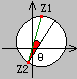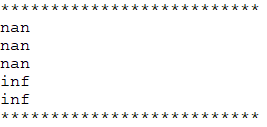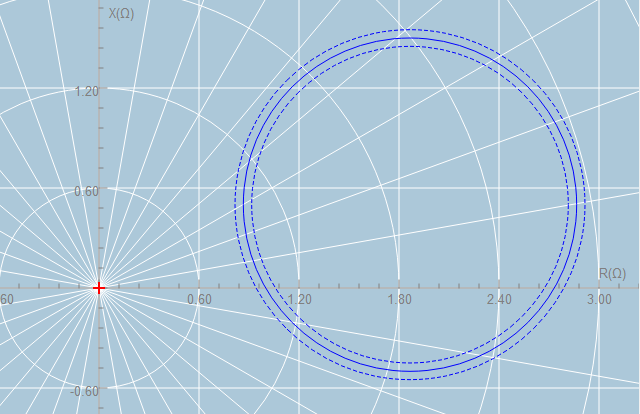# 1.根据圆上的两个点和半径获得两个圆的算法

#define MAXCOM_F(a, b) ((a)-(b)>0.00001) ? true : false
QVector<QRectF> EICWidget::getEllipseCoors(const QPointF &r1, const QPointF &r2, float r)
{
double c1=0.0f, c2=0.0f, A=0.0f, B=0.0f, C=0.0f, x0=0.0f, y0=0.0f, x1=0.0f, y1=0.0f;
if (MAXCOM_F(qAbs(r2.x()-r1.x()),0.0f)) {
c1 = (pow(r2.x(),2)-pow(r1.x(),2)+pow(r2.y(),2)-pow(r1.y(),2))/2/(r2.x()-r1.x());
c2 = (r2.y()-r1.y())/(r2.x()-r1.x());
A = 1.0+pow(c2, 2);
B = 2*(r1.x()-c1)*c2-2*r1.y();
C = pow((r1.x()-c1), 2)+pow(r1.y(), 2)-pow(r, 2);
//如何b^2-4ac <= 0,则赋值为0.0f
double b_ac = sqrt(B*B-4*A*C);
if (qIsNaN(b_ac)) b_ac = 0.0f;
y0 = (-B+b_ac)/(2*A);
x0 = c1-c2*y0;
y1 = (-B-b_ac)/2/A;
x1 = c1-c2*y1;
} else {
float d = sqrt(pow((r2.y()-r1.y()), 2)+pow((r2.x()-r1.x()), 2));
d = sqrt(pow(r, 2)-pow(d/2, 2));
y0 = (r1.y()+r2.y())/2;
x0 = (r1.x()+d);
y1 = (r1.y()+r2.y())/2;
x1 = (r1.x()-d);
}
QVector<QRectF>rectf;
rectf<<QRectF(QPointF(x0-r, y0+r), QPointF(x0+r, y0-r));
rectf<<QRectF(QPointF(x1-r, y1+r), QPointF(x1+r, y1-r));
return rectf;
}

### 在开始写这个计算的时候遇到了几个问题：

• 在最开始没有判断两个点的x相等的情况，这就导致会出现分母为0的情况，使得剩下的的计算毫无意义。

• 没有对b^2-4ac做判断，同样的，当b^2-4ac<0的时候，开根号后结果将为nan，后续的计算也将变得都是错误的。

• 这点是作为提醒自己，当浮点型数据没有赋值后但是没有经过计算的时候，是可以直接进行比较大小相等的，但是在经过计算之后就要在一定的精度内进行比较，不然比较的结果可能是不对的。

### 2.根据圆上的两个点和这两个点连接的线与直径的角度可以确定唯一的一个圆

//根据圆上的两个点和一个倾角确定一个圆
QRectF EICWidget::getEllipseCoor(const QPointF &r1, const QPointF &r2, float ang)
{
//求出两点之间的距离
double d = sqrt(pow((r2.y()-r1.y()), 2)+pow((r2.x()-r1.x()), 2));
//求出圆的半径
double r = qAbs(d/cos(ang*M_PI/180.0f))/2;
//根据两个点和半径求出圆心的坐标
QVector<QRectF> rectfs = getEllipseCoors(r1, r2, r);
//根据角度获取唯一的圆
if (MAXCOM_F(ang, 0.0f)) {
return rectfs.at(0);
} else {
return rectfs.at(1);
}
}### 3.关于浮点型数据计算需要注意的事项：

    qDebug()<<"**************************";
//开方下的值如果小于0.0f则会出现nan
qDebug()<<sqrt(-0.00001);
//如果有一个结果为nan则无论后面是什么运算得到的都将是nan
qDebug()<<1.0+sqrt(-1);
qDebug()<<pow(sqrt(-1), 2);
//分母为0时则会出现inf
qDebug()<<1.0/0.0;
//如果计算的数值过大也会出现inf,
double C = 9999999999999999.0/0.0000000000001;
qDebug()<<pow(C, 100);
qDebug()<<"**************************";• 开方下的值如果小于0，则会出现nan
• 如果在运算的过程中，有一个计算结果为nan则不论后面是什么运算都会出现nan
• 当分母为0时则计算的结果会出现inf
• 如果计算的数值过大也会出现inf

### 4.根据两点和一个偏转角的数据输入：QPointF(1.0f, 1.0f), QPointF(1.0f, 0.0f), 60，得到的图形为（两个虚线圆中的实线为得到的圆形）：08-202644
09-18

10-117391
12-05396
08-092万+
09-08456
12-18363
02-28306
07-202874
08-31401
09-15
06-15907
04-101万+
07-24774
03-171万+
08-06687
09-278336

### “相关推荐”对你有帮助么？

•非常没帮助
•没帮助
•一般
•有帮助
•非常有帮助¥2 ¥4 ¥6 ¥10 ¥20余额支付 (余额：-- )扫码支付获取中扫码支付点击重新获取扫码支付1.余额是钱包充值的虚拟货币，按照1:1的比例进行支付金额的抵扣。
2.余额无法直接购买下载，可以购买VIP、C币套餐、付费专栏及课程。余额充值